Study Guide

# In the Real World

## In the Real World

You might feel like you're taking a foreign language class, calling the same point by polar coordinates (rθ) or rectangular coordinates (xy). Maybe we're talking about a vector, in which case |r| is its magnitude, θ is its direction, and x and y are its components.

The reason for all the choices is that different representations are useful for different tasks. If we want to draw the unit circle,
r = 1 is a much nicer equation than x2 + y2 = 1.

However, if we want to draw a vertical line, x = 2 is much nicer than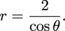Taking things a little closer to the real world, if we're trying to gravel from point A to B in downtown New York we need to use the Manhattan distance to compute the distance. We need to know the x and y components of the vector between points A and B.

However, if we're planning to fly things from point A to point B in the middle of nowhere, we consider the distance as the crow flies. We want to know the straight-line distance from A to B, which is the magnitude of the vector connecting those points.

### Dimensions of Vectors

Vectors aren't always 2-D. We can have an n-dimensional vector for any positive integer n we like. We could have a 100-dimensional vector, or a googolplex-dimensional vector.

We can also have vectors with infinitely many components.

Look at some examples.

Here's a vector with infinitely many components, all of which are 1: (1, 1, 1,...)

We could have a vector where the component in the nth place is n: (1, 2, 3, ...)

We could have a vector whose components alternate between 0 and 1: (0, 1, 0, 1, ...)

### Sample Problem

Is the last component of the vector (0, 1, 0, 1, ...) equal to 0 or a 1?

Sorry—that's a trick question. This vector keeps going forever, it doesn't have a last component.

### Size of Vectors

There are quite a few different ways to measure the size of a vector v = (x1x2, ..., xn).

This sort of measurement is called the taxicab norm or Manhattan distance. For a 2-D vector (x,y), the Manhattan distance

x + y

is the distance we need to travel from the tail of the vector to the head of the vector if we need to stay on the grid-like streets.

We've haven't defined the word norm yet. A norm is a mathematical thing that has to follow a bunch of fussy rules, but if we think of it as a way to measure size, that's close enough. A mathematician may use one norm or another norm depending on what they're doing with their vectors.

In parting, we saw that it's possible to have infinite-dimensional vectors. How could we measure the size of an infinite-dimensional vector?

• ### How to Solve a Math Problem

In three easy steps, we can solve any math problem. And for three easy payments of...

We're not trying to sell you a set of knives that will never dull or a counter top oven that'll cook eggs, bacon and toast all at once. We can at least sell the basic steps to solving every math problem.

There are three steps to solving a math problem.

• Figure out what the problem is asking.

• Solve the problem.

### Sample Problem

Determine all points at which the graph of the parametric function x(t) = -5 + 5t, y(t) = 4and the graph of the parametric function x(t) = 2t + 1, y(t)= tintersect. Round to 5 digits, and use a calculator.

• Figure out what the problem is asking.

The problem is asking for the coordinates of all points (x, y) that occur on both graphs.

Important: If (x, y) occurs on both graphs, it will probably result from different values of the parameter for each graph.

We might want to set the x equations equal and solve

-5 + 5t = 2t + 1

for t, but that will only work if the same value of t generates the point for both graphs. That probably isn't the case.

Instead, an intersection point occurs when we put some value t into the first set of equations and some value s into the second set of equations and find the same point (x, y).

Writing this in symbols, we want

x = -5 + 5t = 2s + 1 and y = 4t = s2.

Dropping the x and y, we want -5 + 5t = 2s + 1 and 4t = s2.

This is a system of two equations with two unknowns, which we know how to solve.

After finding s and t we need to find the coordinates of the point or points (x, y) that occur on both graphs.

• Solve the problem.

We need to solve the system

-5 + 5t = 2s + 1

4t = s2.

Solve the second equation for t: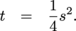Then substitute for t in the first equation: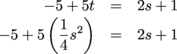This is a quadratic equation. Tidy it so we can use the quadratic formula to solve it.We didn't need to do the last step, where we multiplied through by 4, but it's easier to use the quadratic formula when the coefficients are integers instead of fractions.There are two values of s here that we need to use. To find the appropriate values of x and y we use the second set of equations.

When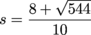we have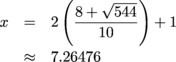andOne point of intersection is approximately
(7.26476, 9.81181).

When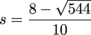we have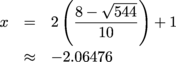andThe other point of intersection is approximately

(-2.06476, 2.34819).

It looks like they run into each other twice, once somewhere around (-2, 2) and once somewhere around (7, 10).
This tells us that we found the correct number of points, and the numbers we found seem to be about right. In practice, this is probably all the checking we would do.

Now we'll show how to check the answers for real.

We'll find the value of t that corresponds to each value of s, and make sure that we find the correct point when we put that value of t into the first set of parametric equations.

Whenwe haveThenandThis agrees with the (x ,y) coordinates we got from the second set of parametric equations, therefore we believe

(7.26476, 9.81181 )

is an intersection point.

Whenwe haveThenandThis agrees with the (x, y) coordinates we got from the second set of parametric equations, so we believe

(-2.06476, 2.34819)

is an intersection point.